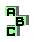PARALLELOGIC TRIANGLES

 r6 Steiner point, first Brocard triangle r185 orthologic triangles, similar triangles r243 Fermat points, Kiepert hyperbola, Steiner dual line, orthic axis, concyclic points r1471 circumcircle, orthologic triangles r1801 centroid, incenter, excenters, circumcenter, extangential triangle r1803 Jerabek hyperbola, orthic triangle r1818 intouch triangle, incircle r1819 orthocenter, circumcenter, altitude feet r1820 altitudes, Euler line r1822 circumcenter, Euler line, orthic triangle r1823 r1912 orthologic triangles, ninecircle